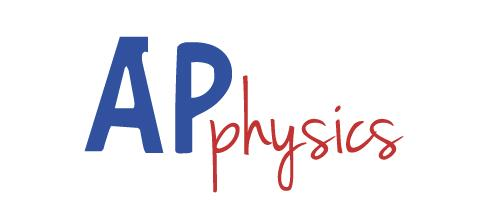# Are You AP Physics Whiz?

8 Questions | Total Attempts: 195SettingsAre you a physics whiz? Think you can complete the following formulas and get all the answers correct? Take this quiz to see how many questions you can answer correctly.

• 1.
The equation for net force is F(net) = m_
• A.

A

• B.

B

• C.

C

• D.

D

• 2.
The equation for the force of friction is F(fric) = μF(_)
• A.

N

• B.

K

• C.

X

• D.

A

• 3.
The equation for torque is τ = r_sinθ
• A.

F

• B.

S

• C.

T

• D.

P

• 4.
The equation for centripetal acceleration is a (_) = v²/r
• A.

B

• B.

C

• C.

D

• D.

E

• 5.
The equation for momentum is  _ = mv
• A.

P

• B.

Q

• C.

R

• D.

S

• 6.
The equation for net force is F(___) = ma
• A.

Net

• B.

Avg

• C.

For

• D.

Tim

• 7.
The equation for the force felt by a charged particle in an electric field is F = _E
• A.

Q

• B.

P

• C.

R

• D.

S

• 8.
The equation for voltage V = I_
• A.

R

• B.

S

• C.

T

• D.

P

Related TopicsBack to top